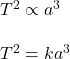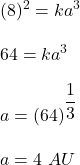Question

From Kepler’s third law, an asteroid with an orbital period of 8 years lies at an average distance from the Sun equal to:
A. 2 astronomical units.
B. 4 astronomical units.
C. 8 astronomical units.
D. 16 astronomical units.
E. It depends on the asteroid’s mass.

1.mocmien2

The correct option is (B).

Explanation:

The Kepler’s third law of motion gives the relationship between the orbital time period and the distance from the semi major axis such that,It is mentioned that, an asteroid with an orbital period of 8 years. So,So, an asteroid with an orbital period of 8 years lies at an average distance from the Sun equal to 4 astronomical units.